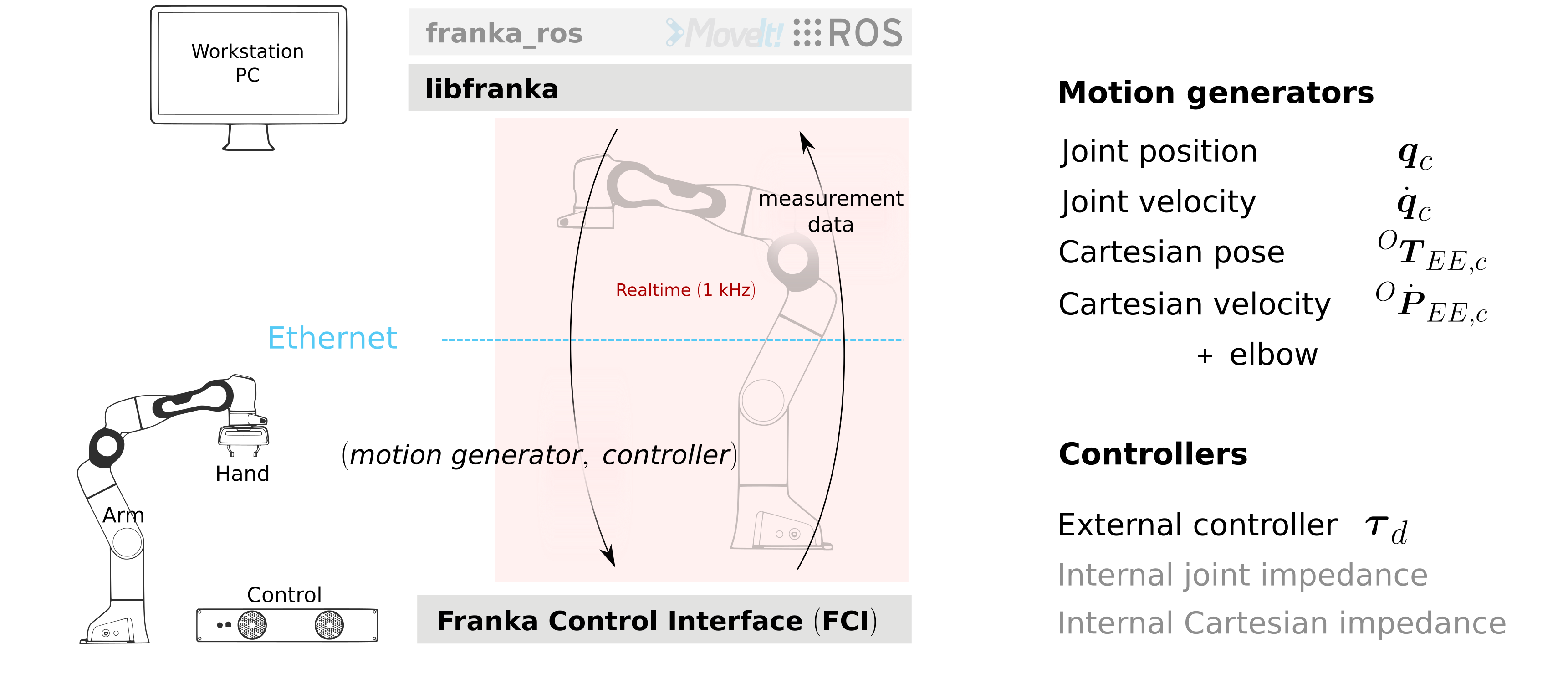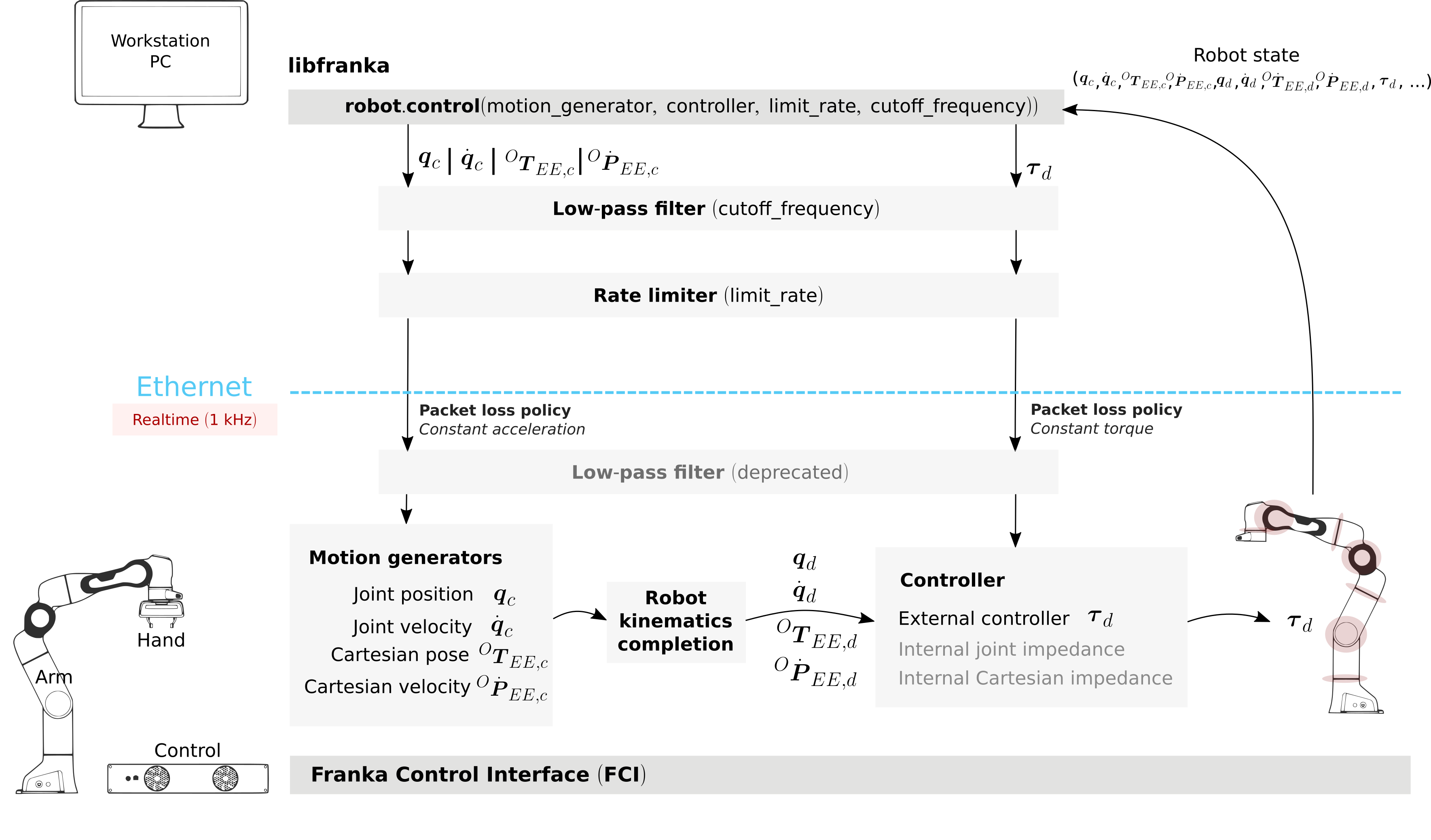# libfranka库¶libfranka 是 FCI 客户端的 C++ 实现。它处理与 Control 的网络通信并提供接口以轻松

• 执行 非实时命令 来控制 Hand 和配置 Arm 参数。
• 执行 实时命令 以运行您自己的 1 kHz 控制回路。
• 读取 机器人状态 以获取 1 kHz 的传感器数据。
• 访问 模型库 以计算期望的运动学和动态参数。

## 非实时命令¶Arm 和 Hand 的非实时命令。

• homing 校准手的最大抓握宽度。
• move, graspstop, 用手移动或抓握。
• readOnce，读取 Hand 状态。

• setCollisionBehavior 它设置接触和碰撞检测阈值。
• setCartesianImpedancesetJointImpedance 为内部笛卡尔阻抗和内部关节阻抗控制器设置阻抗参数。
• setEE 设置从名义末端执行器到末端执行器坐标系的转换 NE_T_EE。从法兰到末端执行器的坐标转换 F_T_EE 的转换分为两个： F_T_NENE_T_EE。从法兰到名义末端执行器的坐标转换 F_T_NE 只能通过管理员界面进行设置。
• setK 设置从末端执行器坐标系到刚度坐标系的坐标变换 EE_T_K
• setLoad 设置负载的动态参数。
• automaticErrorRecovery 清除之前在机器人中发生的任何命令或控制异常。

Arm 或 Hand 上的所有操作（非实时或实时）分别通过 franka::Robotfranka::Gripper 对象执行 。创建对象时，将建立与Arm/Hand的连接：

#include <franka/robot.h>
#include <franka/gripper.h>

...

franka::Gripper gripper("<fci-ip>");
franka::Robot robot("<fci-ip>");


gripper.homing();
robot.automaticErrorRecovery();


## 实时命令¶

• 运动生成器，它定义了关节或笛卡尔空间中的机器人运动。
• 控制器，定义要发送到机器人关节的扭矩。• 只有一个运动发生器，因此使用两个内部控制器之一来跟随命令的运动。
• 只有一个外部控制器，而忽略任何运动发生器信号，即仅转矩控制。
• 一个运动发生器和一个外部控制器，用于在外部控制器中使用 Control 的逆运动学。

std::function<franka::Torques(const franka::RobotState&, franka::Duration)>
my_external_controller_callback;
// Define my_external_controller_callback
...

std::function<franka::JointVelocities(const franka::RobotState&, franka::Duration)>
my_external_motion_generator_callback;
// Define my_external_motion_generator_callback
...

try {
franka::Robot robot("<fci-ip>");
// only a motion generator
robot.control(my_external_motion_generator_callback);
// only an external controller
robot.control(my_external_controller_callback);
// a motion generator and an external controller
robot.control(my_external_motion_generator_callback, my_external_controller_callback);
} catch (franka::Exception const& e) {
std::cout << e.what() << std::endl;
return -1;
}
return 0;
}


robot.control(
[=, &time](const franka::RobotState&, franka::Duration period) -> franka::JointVelocities {
time += period.toSec();

double cycle = std::floor(std::pow(-1.0, (time - std::fmod(time, time_max)) / time_max));
double omega = cycle * omega_max / 2.0 * (1.0 - std::cos(2.0 * M_PI / time_max * time));

franka::JointVelocities velocities = {{0.0, 0.0, 0.0, omega, omega, omega, omega}};

if (time >= 2 * time_max) {
std::cout << std::endl << "Finished motion, shutting down example" << std::endl;
return franka::MotionFinished(velocities);
}
return velocities;
});


// Set joint impedance (optional)
robot.setJointImpedance({{3000, 3000, 3000, 3000, 3000, 3000, 3000}});
// Runs my_external_motion_generator_callback with the default joint impedance controller
robot.control(my_external_motion_generator_callback);
// Identical to the previous line (default franka::ControllerMode::kJointImpedance)
robot.control(my_external_motion_generator_callback, franka::ControllerMode::kJointImpedance);

// Set Cartesian impedance (optional)
robot.setCartesianImpedance({{2000, 2000, 2000, 100, 100, 100}});
// Runs my_external_motion_generator_callback with the Cartesian impedance controller
robot.control(my_external_motion_generator_callback, franka::ControllerMode::kCartesianImpedance);


robot.control([&](const franka::RobotState&, franka::Duration) -> franka::Torques {
return {{0.0, 0.0, 0.0, 0.0, 0.0, 0.0, 0.0}};
});


./examples/generate_joint_velocity_motion <fci-ip>


### 信号处理¶

• 用于平滑用户命令信号的一阶低通滤波器 low-pass filter
• 一个速率限制器 rate limiter，它使用户命令值的时间导数饱和
• 从版本 0.5.0 开始，libfranka 包括一个低通滤波器 low-pass filter，用于 默认运行 的所有实时接口，截止频率为 100 Hz。滤波器平滑命令信号以提供更稳定的机器人运动，但不能防止违反 接口限制

重要

从版本 0.5.0 开始，该 Robot::setFilters 命令已被弃用，以支持该函数的低通滤波器 Robot::control 功能。

• 从版本 0.4.0 开始，所有实时接口的 速率限制器 (rate limiters) 默认运行 。速率限制器 (rate limiters) ，也称为 安全控制器 (safe controllers)，将限制用户发送的信号的变化率，以防止违反 接口限制。. 对于运动发生器，它将限制加速度和加加速度，而对于外部控制器，它将限制扭矩率。它们的主要目的是提高控制回路的稳健性。在数据包丢失的情况下，即使您发送的信号符合接口限制，Control 也可能检测到违反速度、加速度或加加速度限制的情况。速率限制将调整您的命令以确保不会发生这种情况。有关更多详细信息，请查看 不合规错误部分

警告

速率限制将确保除了逆向​​运动学之后的关节限制之外没有任何限制被违反，其违反会产生以 cartesian_motion_generator_joint_* 开头的一系列错误。有关更多详细信息，请查看 不合规错误部分

提示

速率限制器中使用的限制在 franka/rate_limiting.h 接口限制中定义并设置。如果这会产生抖动或不稳定的行为，您可以将限制设置为较低的值，激活低通滤波器或降低其截止频率。

// Set Cartesian impedance (optional)
robot.setCartesianImpedance({{2000, 2000, 2000, 100, 100, 100}});
// Runs my_external_motion_generator_callback with the Cartesian impedance controller,
// rate limiters on and low-pass filter with 100 Hz cutoff
robot.control(my_external_motion_generator_callback, franka::ControllerMode::kCartesianImpedance);
// Identical to the previous line (default true, 100.0 Hz cutoff)
robot.control(my_external_motion_generator_callback, franka::ControllerMode::kCartesianImpedance, true, 100.0);
// Runs my_external_motion_generator_callback with the Cartesian impedance controller,
// rate limiters off and low-pass filter off
robot.control(my_external_motion_generator_callback, franka::ControllerMode::kCartesianImpedance, false, 1000.0);


// With rate limiting and filter
robot.control(my_external_controller);
// Identical to the previous line (default true, 100.0 Hz cutoff)
robot.control(my_external_controller, true, 100.0);
// Without rate limiting but with low-pass filter (100.0 Hz)
robot.control(my_external_controller, false);
// Without rate limiting and without low-pass filter
robot.control(my_external_controller, false, 1000.0);


### 蕴含的更深层次¶• 数据包丢失，如果您：

• 由于您的 PC 和 网卡的性能，没有很好的网络连接。
• 您的控制回路计算时间太长（您可以有，取决于您的网卡和 PC 配置，大约 < 300 $$\mu s$$ 的时间用于您自己的控制回路）。

在这种情况下，Control 假设一个恒定加速度模型或一个恒定扭矩来推断您的信号。如果 >=20 个数据包连续丢失，则控制循环将停止，并伴随 communication_constraints_violation 异常的抛出。

• 一个可选的低通滤波器 low-pass filter。您可以使用非实时命令设置截止频率 setFilters。将其设置 1000 为禁用它。从版本 0.5.0 起它的使用已被弃用。

## 机器人状态¶

• 关节层级信号：点击和估计的关节角度及其导数、关节扭矩及其导数、估计的外部扭矩、关节碰撞/接触。
• 笛卡尔层级信号：笛卡尔位姿、配置的末端执行器和负载参数、作用在末端执行器上的外部扭力、笛卡尔碰撞
• 接口信号：上一小节中解释的最后命令和期望值及其导数值。

franka::RobotState state = robot.readOnce();


size_t count = 0;
// Printing to std::cout adds a delay. This is acceptable for a read loop such as this,
// but should not be done in a control loop.
std::cout << robot_state << std::endl;
return count++ < 100;
});


## Model库¶

• 所有机器人关节的正向运动学。
• 所有机器人关节的体矩阵和零雅可比矩阵。
• 动态参数：惯性矩阵、科式力和离心力矢量和重力矢量。

## 错误¶

### 由于不合规的命令值而导致的错误¶

• 由于 运动发生器的错误初始值 (wrong initial values of a motion generator) 而导致的错误：
• joint_motion_generator_start_pose_invalid
• cartesian_position_motion_generator_start_pose_invalid
• cartesian_motion_generator_start_elbow_invalid
• cartesian_motion_generator_elbow_sign_inconsistent

double time{0.0};
robot.control(
[=, &time](const franka::RobotState& robot_state, franka::Duration period) -> franka::JointPositions {
time += period.toSec();
if (time == 0) {
// Send the last commanded q_c as the initial value
return franka::JointPositions(robot_state.q_c);
} else {
// The rest of your control loop
...
}
});

• 由于使用关节位置/速度运动生成器违反 位置限制 而导致的错误，这将产生 joint_motion_generator_position_limits_violation 报错. 解决此错误应该很简单：确保您发送的值在 限制值 范围内。笛卡尔接口对逆运动学计算之后得到的关节信号也有限制：如果 Control 的逆运动学求解器产生超出限制的某个关节配置，cartesian_motion_generator_joint_position_limits_violation 将被触发。
• 由于违反 速度 (velocity) 的限制产生的错误和不连续的错误，其指的违反 加速度 (acceleration) 和/或 加加速度 (jerk) 的限制。如果您使用关节运动生成器，则可能的错误是
• joint_motion_generator_velocity_limits_violation
• joint_motion_generator_velocity_discontinuity （违反加速度限制）
• joint_motion_generator_acceleration_discontinuity （违反加加速度限制）

• 笛卡尔限制

• cartesian_motion_generator_velocity_limits_violation
• cartesian_motion_generator_velocity_discontinuity （违反加速度限制）
• cartesian_motion_generator_acceleration_discontinuity （违反加加速度限制）
• 逆运动学解算后的关节限制

• cartesian_motion_generator_joint_velocity_limits_violation
• cartesian_motion_generator_joint_velocity_discontinuity （违反加速度限制）
• cartesian_motion_generator_joint_acceleration_discontinuity （违反加加速度限制）

• 速度 $$\dot{q}_{c,k} = \frac{q_{c,k} - q_{c,k-1}}{\Delta t}$$
• 加速度 $$\ddot{q}_{c,k} = \frac{\dot{q}_{c,k} - \dot{q}_{c,k-1}}{\Delta t}$$
• 加加速度 $$\dddot{q}_{c,k} = \frac{\ddot{q}_{c,k} - \ddot{q}_{c,k-1}}{\Delta t}$$

• controller_torque_discontinuity

Control 还使用反向欧拉法计算扭矩率，即 $$\dot{\tau}_{d,k} = \frac{\tau_{d,k} - \tau_{d,k-1}}{\Delta t}$$. 用户先前期望的扭矩也在机器人状态下作为 $$\tau_d$$ 发回。因此，即使在数据包丢失的情况下，您也可以提前计算产生的扭矩率。

libfranka 版本中包含的速率限制器从 0.4.0 版本起修改用户发送的信号，使它们符合所有这些限制，除了逆向运动学之后的关节限制。您可以查看 include/franka/rate_limiting.hsrc/rate_limiting.cpp 以获取有关如何计算由此限制的所有接口的速度、加速度和加加速度的示例代码。我们再次强调，在不连续信号上使用速率限制很容易产生不稳定行为，因此在启用此 稳健性 robustness 功能之前，请确保您的信号足够平滑。

### 行为错误¶

• 反射性错误 Reflex errors。如果估计的外部扭矩 $$\hat{\tau}_{ext}$$ 或力 $${}^O\hat{F}_{ext}$$ 超过配置的阈值，将分别触发 cartesian_reflexjoint_reflex 错误。您可以使用 franka::Robot::setCollisionBehavior 非实时命令配置阈值。

提示

如果您希望机器人与环境接触，您必须将碰撞阈值设置为更高的值。否则，一旦你抓住一个物体或推向一个表面，就会触发反射错误。此外，如果阈值较低，在没有接触的情况下非常快速或突然的运动可能会触发反射错误；外部扭矩和力只是估计值 estimated，根据机器人配置它们可能不准确，尤其是在高加速阶段。您可以监视它们的值通过观察机器人状态中的 $$\hat{\tau}_{ext}$$$${}^O\hat{F}_{ext}$$

• 自碰撞避免 Self-collision avoidance。如果机器人达到接近自碰撞的配置，则会触发 self_collision_avoidance_violation 错误。

警告

此错误并不能保证机器人在任何配置和速度下都能防止自碰撞。如果使用扭矩接口以全速驱动机器人，机器人可能会发生自我碰撞。

• 如果达到 扭矩传感器限制tau_j_range_violation 错误将被触发。这并不能保证传感器在任何高扭矩相互作用或运动后不会损坏，但旨在防止其中一些。

• 如果达到 最大允许功率power_limit_violation 错误将触发并阻止机器人在控制回路期间停止和接合锁止装置。

• 如果您达到关节或笛卡尔极限，您将分别得到一个 joint_velocity_violation 或一个 cartesian_velocity_violation 错误